# Whittaker equation

(diff) ← Older revision | Latest revision (diff) | Newer revision → (diff)
Jump to: navigation, search

A linear homogeneous ordinary differential equation of the second order: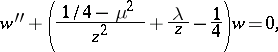(*)

where the variables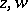and the parametersmay take arbitrary complex values. Equation (*) represents the reduced form of a degenerate hypergeometric equation and was first studied by E.T. Whittaker . Forthe Whittaker equation is equivalent to the Bessel equation. If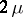is not an integer, a fundamental system of solutions of the Whittaker equation consists of the functionsand; here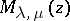is the Whittaker function (cf. Whittaker functions). For any value of the parameters the general solution of the Whittaker equation may be written in the form of a linear combination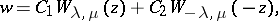where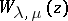is the Whittaker function.